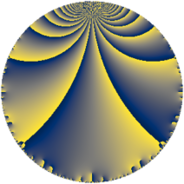# Properties

 Label 126.4.gLevel $126$ Weight $4$ Character orbit 126.g Rep. character $\chi_{126}(37,\cdot)$ Character field $\Q(\zeta_{3})$ Dimension $20$ Newform subspaces $7$ Sturm bound $96$ Trace bound $5$

# Related objects

## Defining parameters

 Level: $$N$$ $$=$$ $$126 = 2 \cdot 3^{2} \cdot 7$$ Weight: $$k$$ $$=$$ $$4$$ Character orbit: $$[\chi]$$ $$=$$ 126.g (of order $$3$$ and degree $$2$$) Character conductor: $$\operatorname{cond}(\chi)$$ $$=$$ $$7$$ Character field: $$\Q(\zeta_{3})$$ Newform subspaces: $$7$$ Sturm bound: $$96$$ Trace bound: $$5$$ Distinguishing $$T_p$$: $$5$$

## Dimensions

The following table gives the dimensions of various subspaces of $$M_{4}(126, [\chi])$$.

Total New Old
Modular forms 160 20 140
Cusp forms 128 20 108
Eisenstein series 32 0 32

## Trace form

 $$20q - 40q^{4} - 18q^{5} - 8q^{7} + O(q^{10})$$ $$20q - 40q^{4} - 18q^{5} - 8q^{7} + 8q^{10} + 6q^{11} - 192q^{13} - 72q^{14} - 160q^{16} - 150q^{17} + 46q^{19} + 144q^{20} + 256q^{22} + 210q^{23} - 68q^{25} - 120q^{26} + 40q^{28} - 336q^{29} - 138q^{31} - 512q^{34} - 426q^{35} - 242q^{37} - 192q^{38} + 32q^{40} + 2016q^{41} + 1288q^{43} + 24q^{44} + 40q^{46} + 102q^{47} + 1412q^{49} - 1824q^{50} + 384q^{52} - 1182q^{53} - 1916q^{55} + 288q^{56} - 136q^{58} + 90q^{59} - 294q^{61} + 3744q^{62} + 1280q^{64} + 1524q^{65} + 1226q^{67} - 600q^{68} - 1520q^{70} - 672q^{71} + 1142q^{73} - 312q^{74} - 368q^{76} - 2226q^{77} + 1266q^{79} - 288q^{80} + 1200q^{82} + 2568q^{83} - 4892q^{85} - 984q^{86} - 512q^{88} - 2754q^{89} + 2100q^{91} - 1680q^{92} - 216q^{94} - 1974q^{95} - 160q^{97} + 2880q^{98} + O(q^{100})$$

## Decomposition of $$S_{4}^{\mathrm{new}}(126, [\chi])$$ into newform subspaces

Label Dim. $$A$$ Field CM Traces $q$-expansion
$$a_2$$ $$a_3$$ $$a_5$$ $$a_7$$
126.4.g.a $$2$$ $$7.434$$ $$\Q(\sqrt{-3})$$ None $$-2$$ $$0$$ $$-15$$ $$35$$ $$q+(-2+2\zeta_{6})q^{2}-4\zeta_{6}q^{4}+(-15+\cdots)q^{5}+\cdots$$
126.4.g.b $$2$$ $$7.434$$ $$\Q(\sqrt{-3})$$ None $$-2$$ $$0$$ $$-6$$ $$-7$$ $$q+(-2+2\zeta_{6})q^{2}-4\zeta_{6}q^{4}+(-6+6\zeta_{6})q^{5}+\cdots$$
126.4.g.c $$2$$ $$7.434$$ $$\Q(\sqrt{-3})$$ None $$-2$$ $$0$$ $$7$$ $$-20$$ $$q+(-2+2\zeta_{6})q^{2}-4\zeta_{6}q^{4}+(7-7\zeta_{6})q^{5}+\cdots$$
126.4.g.d $$2$$ $$7.434$$ $$\Q(\sqrt{-3})$$ None $$2$$ $$0$$ $$-9$$ $$-28$$ $$q+(2-2\zeta_{6})q^{2}-4\zeta_{6}q^{4}+(-9+9\zeta_{6})q^{5}+\cdots$$
126.4.g.e $$4$$ $$7.434$$ $$\Q(\sqrt{-3}, \sqrt{193})$$ None $$-4$$ $$0$$ $$7$$ $$6$$ $$q-2\beta _{2}q^{2}+(-4+4\beta _{2})q^{4}+(\beta _{1}+3\beta _{2}+\cdots)q^{5}+\cdots$$
126.4.g.f $$4$$ $$7.434$$ $$\Q(\sqrt{-3}, \sqrt{193})$$ None $$4$$ $$0$$ $$-7$$ $$6$$ $$q+2\beta _{2}q^{2}+(-4+4\beta _{2})q^{4}+(-\beta _{1}+\cdots)q^{5}+\cdots$$
126.4.g.g $$4$$ $$7.434$$ $$\Q(\sqrt{-3}, \sqrt{1345})$$ None $$4$$ $$0$$ $$5$$ $$0$$ $$q+2\beta _{2}q^{2}+(-4+4\beta _{2})q^{4}+(\beta _{1}+2\beta _{2}+\cdots)q^{5}+\cdots$$

## Decomposition of $$S_{4}^{\mathrm{old}}(126, [\chi])$$ into lower level spaces

$$S_{4}^{\mathrm{old}}(126, [\chi]) \cong$$ $$S_{4}^{\mathrm{new}}(7, [\chi])$$$$^{\oplus 6}$$$$\oplus$$$$S_{4}^{\mathrm{new}}(14, [\chi])$$$$^{\oplus 3}$$$$\oplus$$$$S_{4}^{\mathrm{new}}(21, [\chi])$$$$^{\oplus 4}$$$$\oplus$$$$S_{4}^{\mathrm{new}}(42, [\chi])$$$$^{\oplus 2}$$$$\oplus$$$$S_{4}^{\mathrm{new}}(63, [\chi])$$$$^{\oplus 2}$$